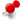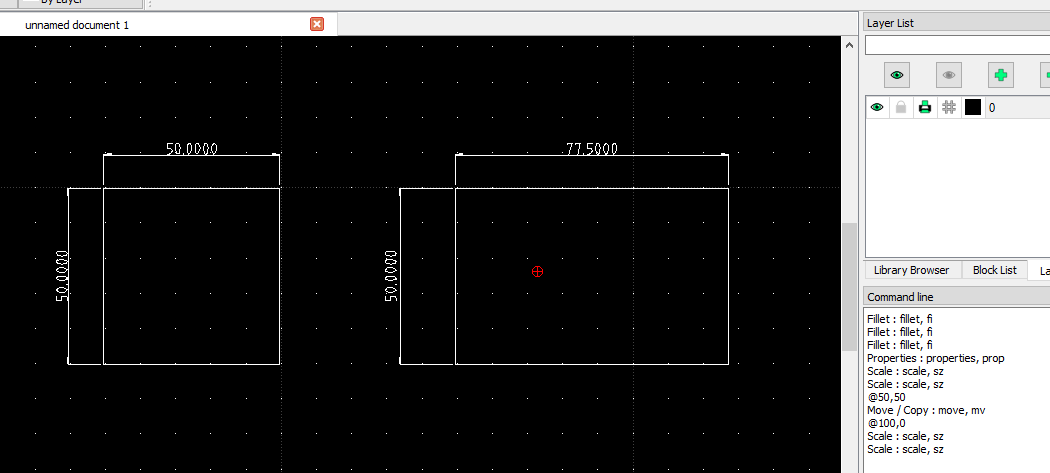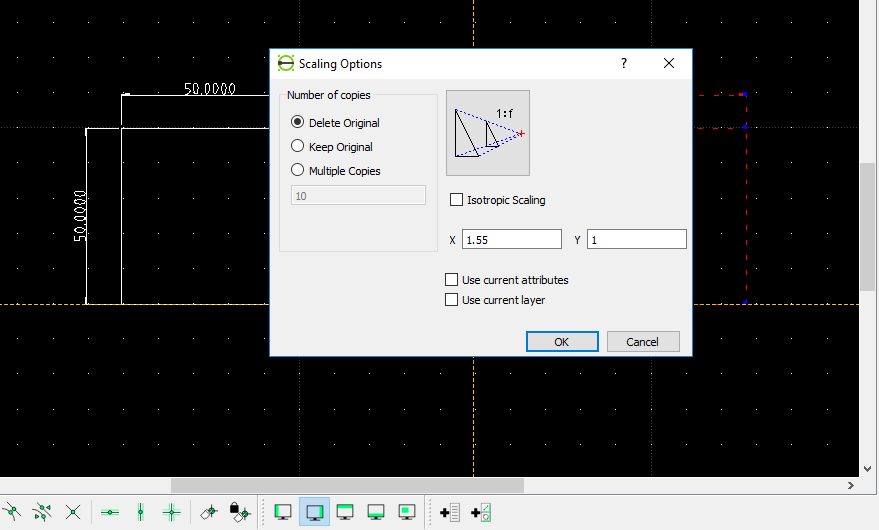# How do you create an object with specific dimensions?ClassicListThreaded3 messagesOpen this post in threaded view
|

## How do you create an object with specific dimensions?

 I'd like to create a square of specific dimensions but don't see how to manually change the length or width to an exact number
 In reply to this post by CGannon Hi CGannon, There is a way to change an object to a specific dimension by using the SCALE tool.The screen shot above illustrates the before and after objects.  First I used the RECTANGLE tool to create a square with the dimensions of 50 x 50.  Then I made a copy of it.  So now I have two 50 x 50 squares.  Next I selected the square I wanted to change the dimensions of.  In this case the square on the right.  I selected both the square and the dimensions.  Next I picked the SCALE tool.  When asked for a reference point I selected the lower left hand corner.  Then the following window popped up.I unchecked the box labeled "Isotropic."  (an object that has the same value when measured in different directions.) Now for some math.  Take the dimension you want to change to and divide by the existing dimension.  For this example I wanted to increase the width to 77.5 units.  77.5/50 = 1.55.  I entered 1.55 into the X box and left the Y box at one.  Hit enter and you can see the result. This works for both X and Y.  Use this same procedure if you want to go smaller.  To get a X value of 46.375: 46.375/50 = 0.8675. If you want to keep the X/Y ratios the same then check the Isotropic box.  If you want to double the size of an object, make sure the Isotropic box is checked, enter 2 in the X box and hit enter.  For my box the size would then be 100 x 100.  .5 would half the size: 25 x 25. Hope this helps.